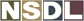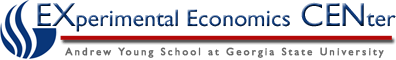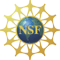Students / Subjects# Calculating Percentage Change

What does the "percentage change" () element of our elasticity formula mean? We simply want to look at how much the quantity and price changes, and then express this as a percentage. It is important to note that there are two common ways that percentage change is calculated. The two different methods give slightly different answers, so it is important to know which method you are being asked to use. Both methods are presented here; the standard method and the mid-point method.

Standard Method= New - OldOld100

We can use this formula to calculate the percentage change between any two numbers or quantities. As applied to economics, we typically present percentage change as follows where...

P= New Price
P= Old Price
Q= New Quantity
Q= Old Quantity
Y= New Income
Y= Old Quantity

Percentage change in price:price = (P- P)P100

Percentage change in quantity:quantity = (Q- Q)Q100

Percentage change in income:income = (Y- Y)Y100

Example

Suppose the price of Atlanta Thrashers tickets falls from \$45 to \$40 and the quantity demanded for tickets increases from 18,000 tickets to 23,000 tickets. Find the price elasticity of demand.price = (40 - 45)45100

= 11%quantity = (23,000 - 18,000)18,000100

= 28%

Now that we have calculated our percentage change in price and quantity demanded, we can measure the price elasticity of demand.

E=change in quantity demandedChange in Price

E= 11%28%

E= 39%

Midpoint Method

Calculating percentage change as done above is often sufficient. However, you may notice that if we calculate the percentage change in price as (45 - 40)/40 x 100 we find that the percentage change is (-12.5 percent). In other words, it makes a difference if we look at the change as a rise or a fall; this is "end-point problem". To get around this problem, economists use the average of the two values as shown in the formulas below.price = (P- P).5(P+ P)100

This formula is not as complicated as it may look; the only thing we have added is finding the average in the numerator to take care of the "end-point problem"..5(P+ P)This gives us an average of the two values; we can use this for any two values we may need to average including price, quantity, and income.

Example

To demonstrate how to find percentage change using the mid-point method, consider the following situation. Bill's income increased from \$100,000 to \$108,000 and his consumption of cruises increased from one cruise to two. Find the Income Elasticity of Demand.quantity = (2 - 1).5(1 + 2)100 = 67%income = (\$108,000 - \$100,000).5(\$100,000 + \$108,000)100 = 8%

Income Elasticity= 67%8%

= 8.4